Open in App
Not now

# What is Gravity?

• Last Updated : 22 Aug, 2022

There is a story about the discovery of gravity which says that Newton discovered gravity in the year 1665 when he was sitting under the apple tree, and suddenly an apple fell on his head, which led him to the question about falling objects. He questioned himself why all objects fall and also questions that does the moon also fall ? all these questions led him to discover gravity and the law of universal gravitation. Newton’s gravity works very well in everyday life, but it does not account for time, or it fails when we go faster compared to the speed of light, which was later 1915 modified by Albert Einstein in his brand new theory general theory of relativity. Which works very well in all the situations that Newton’s theory could not explain. Examples of gravity are,

1. An object falling on the planet is due to the gravitational pull exerted by the planet (it is not only the planet pulling the object but also the object pulling the planet’s gravity is exerted by both the bodies.
2. Planets revolve around the sun, or the moon revolves around the earth. This is due to the gravitational pull exerted by the sun and the earth, respectively.
3. Satellites, clusters, stars, galaxies, and the clusters of galaxies are influenced by gravity.

## Classical or Newtonian view of gravity

According to the Newtonian view, gravity is a force that acts between bodies that have mass, and it is given by the formula,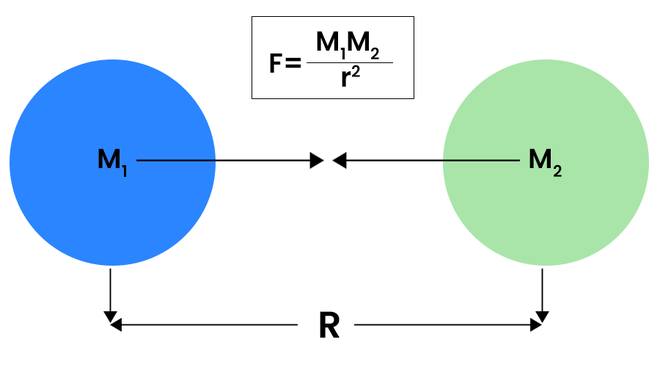F = GM1M2/r2

Where,

• F = forces between them
• G = universal gravitational constant
• M1 M are masses of the  bodies and
• r = distance between them

### What does really gravity do?

Gravity is the reason behind the moon revolving around the earth or satellites revolving around earth, and also the earth revolving around the sun so we can say it acts universally. All the celestial bodies which have a mass encounter another mass (body), then gravity will act upon them, and strength will, of course, depend on their respective masses and the distance between them.

Comparing newtons second law and gravity formula

F = M1a ⇢ (1)

F = GM1M2/r2 ⇢ (2)

M1 = mass of the first object

a = acceleration of an object produced when force F is applied in this case the gravitational force

Equating both the equation we get,

a = GM2/r2

### Gravitational field

The gravitational field around an object is given by the formula,

g = Gm/r

Where,

• g = Gravitational field
• G = Universal gravitational constant
• r = Distance

This means that gravity is greater where a body is more massive and has a smaller radius. This is why even though mars is 1/10th of its mass that of the earth, the gravitational pull around mars is greater than 1/10th  that of earth.

Question: Calculate the gravitational field (gravitational acceleration) of an object having mass 5 times that of the earth. (g of earth = 9.8 m/s2, radius of earth = 6.3 × 10 m, G = 6.67 × 10-11 m = 6 × 1024 kg)

Solution:

Gravitational acceleration is given by,

g = Gm/r2

Then,

g = (6.67 × 10-11 ) × 5(6 × 1024 )/(6.3 × 106)

g = 49 m/s2

Here we can know that gravitational acceleration is just in the ratio of mass. If we know the g of any object and its mass, then the gravitational acceleration of another body with a given ratio mass of the known body then we can easily determine the gravitational acceleration of the unknown body

### Escape velocity

Gravity also affects the escape speed (it is the minimum speed required to escape the surface of the planet) of the objects higher the gravitational pull of an object greater the velocity required to escape the planet.

v = √(Gm1m2/r)

m1, m = masses of the body

Or

v = √(2gr)

Here,

• v = velocity required to escape the surface.
• g = gravitational acceleration.
• r = distance from the center.

Question: Calculate the escape velocity of mars, given its mass, is 1/10th that of the earth. (g = 9.8 m/s2, radius of mars = 3.3 × 106 m)

Solution:

We know that,

v = √2gr

Then putting all the values,

v = √2(g/10)r

v = 5.03 km/s

Extent of gravity

Here in the Newtonian formula, force is directly proportional to masses,

F ∝ M1M2

This means if masses of bodies keep on increasing, the action of gravity too will increase

Black holes: black holes are the best examples of to which extent gravity can act. Black holes are large mass objects compact within a small volume. Due to this, their gravitational pull is so strong that it swallows all the objects coming through it (near the event horizon). Even light cannot escape from it.

### General theory of relativity or Einstein’s view on gravity

Einstein, in his general theory of relativity, describes gravity from a different perspective. In his theory, he made some statements like nothing can travel faster than light. Literally, means nothing. Einstein imagined, what would happen if we somehow managed to vanish the sun or displace from its position, then according to Newtonian gravity, the effect of this event would be instant means as vanish the sun, the earth’s position will change instantly, i.e., it will deflect from its orbit.

According to the general theory of relativity, nothing can travel faster than light, even information, so according to this theory, the earth will get information about the sun’s absence in nearly 8 minutes and 21 seconds later because it takes light this much time to reach earth from the sun .so the information carrying sun’s absence will take at least this much time to reach earth.

### The fabric of space-time

Newton treated space and time as separate quantities, but Einstein unified them together into space-time and gave his own theory about gravity. According to general relativity, gravity is not a force, or we can say it does not fit with our intuition of force. Rather, gravity is just a curvature in the space-time fabric. To understand it, we can say it is analogous to cloth of fabric analogous to space-time fabric. If we somehow fixed the cloth of fabric from all corners in the air, then if we place an object having mass to its center, what will happen will warp or make curvature in the fabric, and if we place another small mass object in the fabric what will happen, it will slide toward center due curvature made by a big mass object placed at the center in the fabric.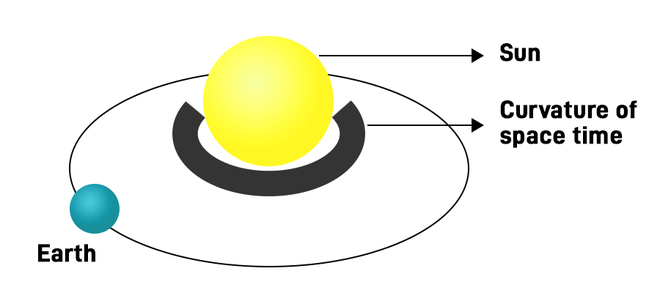This whole situation may be considered analogous to our solar system, except the planets do not fall in the sun, but this gives a small glimpse of understanding Einstein’s theory of gravity. To understand more, we need to think about how an object travels in space(or space-time). According to Newton’s second law, an object will remain at rest if it is at rest, or it will move with uniform velocity in a straight line if it is moving till an external force acts on it. But what if the straight line it is following is curved or appears to be curved to an external observer, that is what happens to mass (or say) earth here.

Due to the mass of the earth, the space around it is curved, due to which the objects moving with uniform velocity in a straight line (in its reference frame) appears to change their direction due to the curvature of space-time in the presence of earth for an external observer. Earth doesn’t pull objects it is due to the curvature that objects fall into the earth or revolve around it. And it does not accelerates it that doesn’t seem familiar, but this theory tells exactly this.

So there is a big difference between Newtonian and Einstein’s views on gravity. In the Newtonian picture of gravity, the objects falling into the surface of the earth accelerate, while in Einstein’s view, it is just following its straight-line inertial path, and according to Newtonian gravity, the earth pulls the objects or exerts a force, but in Einstein’s view the object is just following its straight-line inertial path which appears as curved to an external observer due to the curvature of space-time.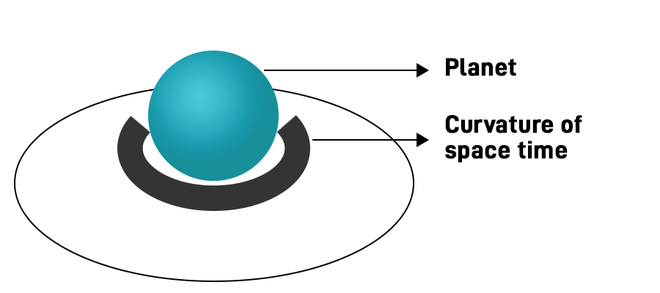Let’s imagine a man falling off the roof of a house. We can clearly see that he will not feel his own weight, i.e., no force that is just like a man in space standing still means they are not just equivalent. They are the same thing, just one in open space is not in curved space, but another is in curved space, that’s why it appears to be falling.

The man in the space also doesn’t feel his own weight, i.e., no force acting on it, and he would be the perfect example of an inertial observer that is not under any influence of force and neither accelerating and if you want to measure the acceleration of the man falling you will need an inertial observer. Sad to say, but no, remember, according to Newtonian gravity, the downward force is acting on you that is gravity, and it is balanced by the normal force acting on you upward, but we know that gravity is not a force, so the only force acting on you is upward(normal force), and we know that if the net force acting on us is not zero, then we cannot be an inertial observer net force acting on you should be zero, and to be amazed we here rest on earth accelerating every time seems ambiguous but imagine to measure our acceleration we need someone who is in  inertial reference frame like someone in space or man falling from the roof because no force is acting on them and neither they are accelerating.

### Bending of light due to gravity

gravity also affects the light in the way by changing its path or bending the light. If some massive object is kept between a star whose light is coming to earth and whose path is visible from the earth, it seems to have a  different position due to the bending of light due to the gravitational influence of the body in between. In fact, this was the first method to test general relativity; during world war 2, Arthur Eddington and some of his companions performed a series of measurements during a total solar eclipse that time and found that the position of the star was deflected exactly the same amount as predicted by general relativity.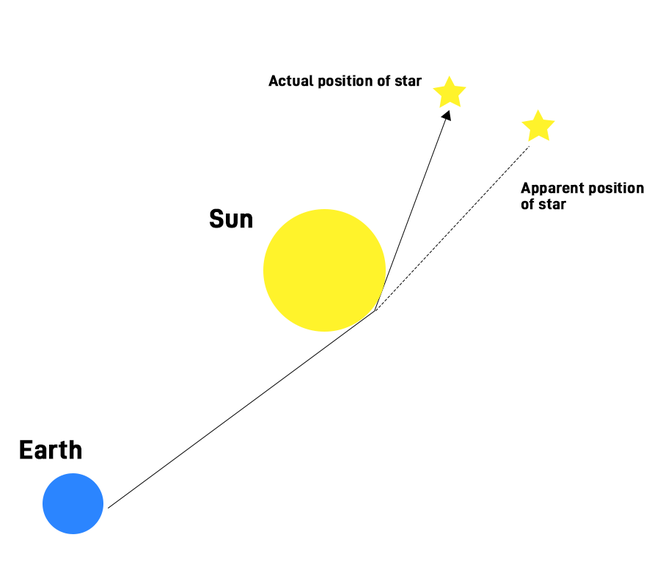### Gravitational lensing

Gravitational lensing is another effect due to gravity, or a more advanced effect of bending of light here light coming is so distorted that we can use multiple images of the same star .and this can easily be observed by our telescopes .and this also testifies the Einstein’s theory of gravity.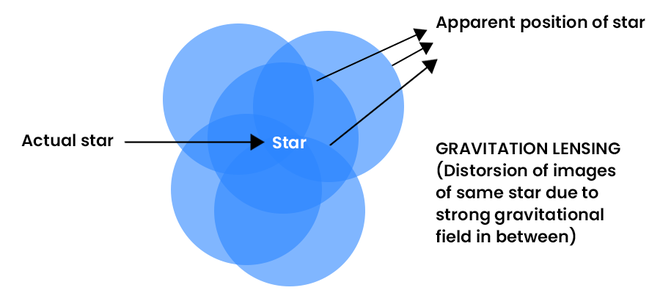### Sample Questions

Question 1: What is the force between two objects having a mass of object A 5 × 1022 kg and object B with a mass of 3.4 × 1011 and they are 20 km apart? (G = 6.6743 × 10-11 m3 kg-1 s-2)

Solution:

A = 51 × 1022 Kg,

B = 3.4 × 1011 Kg

r = 20000 m

We know that gravitational force between two objects is,

F = Gm1m2/r2

Putting all values,

F = 28.34 × 1014  N

Question 2: What is the difference between Newtonian gravity and Einstein’s view on gravity?

The most common difference is the time, in Newtonian gravity, there is, as such, no description of how gravity works through time but in Einstein’s gravity, there is a good description of gravity through time. And that is why Nowadays Einstein’s theory of gravity is widely accepted, and also the GPS (global positioning systems) are made possible due to our understanding of gravity through time

Question 3: If somehow the sun is made to disappear from its place, how much time will we get to know the absence of the sun?

Since we know that nothing can travel faster than light as we learned in this article,we can surely say that if the information of absence of sun tries to reach the earth with the fastest speed possible (that is the speed of light) it will take at least 8 minutes and 23 seconds to reach the earth (light takes this much time to reach the earth), (distance of the earth from the sun is 15 crore kilometers and speed of light is c = 3 × 108 m/s).

Question 4: If 1000 N force is acting between two bodies with a mass of 1000 kg and 6.67 × 10-11 kg, then what is the distance between two bodies?

Solution:

Force = 1000 N,

A = 1000 kg,

b = 6.67 × 10-11 kg

r = ?

We know that.

F = Gm1m2/r2

r2 = (6.67 × 10-11) × (6.67 × 10-11)/1000

r = 6.67 × 10-11m

Question 5: If a planet is 100 crore km away from earth and also has a significant influence of gravity, and if that planet is displaced from its position to somewhere else, then after how much time here on earth we will get two to know the absence of planet?

Solution:

We know that the fastest way that a planet can send the signal of its absence is the speed of light which is 3 × 10 m/s, and the distance given is 100 crore km or 1012 m,

So the time taken is 1012/3 × 108

T = 3.333 × 103 S

My Personal Notes arrow_drop_up
Related Articles